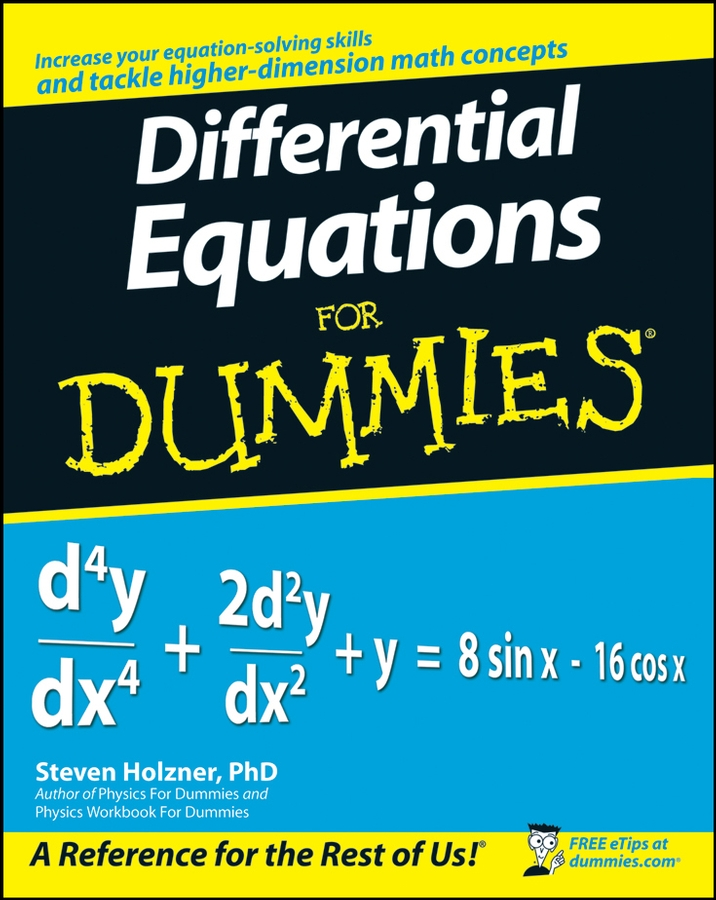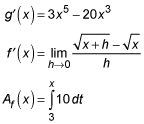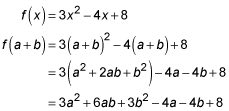##### Differential Equations For DummiesA function is basically anything you can graph on your graphing calculator in “y =” or graphing mode. The line y = 3x – 2 is a function, as is the parabola y = 4x2 – 3x + 6. On the other hand, the sideways parabola x = 5y2 + 4y – 10 isn’t a function because there’s no way to write it as y = something. (Unless you write y = ± something, which doesn't count.)

You can determine whether or not the graph of a curve is a function with the vertical line test. If there’s no place on the graph where you could draw a vertical line that touches the curve more than once, then it is a function. And if you can draw a vertical line anywhere on the graph that touches the curve more than once, then it is not a function.

You can rewrite the above functions using f(x) or g(x) instead of y. This changes nothing; using something like f(x) is just a convenient notation. Here’s a sampling of calculus functions:Virtually every single calculus problem involves functions in one way or another. To review some function basics, try the following examples.

## Practice questions

1. If f(x) = 3x2 – 4x + 8, what does f(a + b) equal?
2. For the line g(x) =